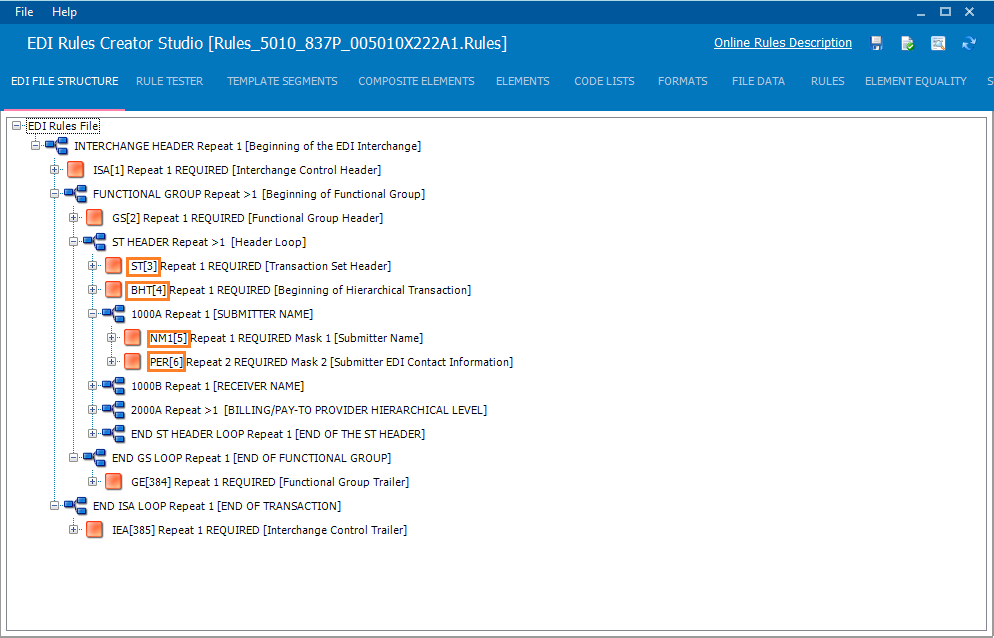# Segment Ordinals

### Segment Ordinals

Ordinal numbers are used throughout the EDI rules file in order to identify segments, elements and composite elements. By using a segment’s ordinal number we can add rules, accepted values, etc.

### Segment Ordinal Number

A Segment Ordinal Number is just the position of the segment in the EDI hierarchy structure. Segment ordinal numbers are automatically displayed next to the segment name.We can see from the picture above that segment ST has a segment ordinal number of 3 and segment BHT has a segment ordinal of 4. Every segment has an ordinal number. It does not matter how many times a segment repeats or how many times the loop containing the segment repeats, the segment’s ordinal number is always the same.

### Element Ordinal Number

An Element Ordinal Number is the position of an element in a segment starting with position 1.

### Example

SEG*A*B*C*D~

We can see from the above example that segment SEG has 4 elements; A,B,C,D. The element ordinal position of element A is 1 and the element ordinal position of element D is 4.

### Composite Ordinal Number

A Composite Ordinal Number is the position of a composite element in a data element. It follows the same rules as the element ordinal number.

in Creating EDI Rules Files